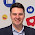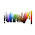## Saturday, 26 May 2012

### 3DQR

Emart in Korea just came up with something amazing; a sundial-like sculpture where the shadows make, between 12 and 1, a QR code you can scan to get info about special offers. I had to have a go myself!
This is a 3D rendering of a 3D shape which, when the light is from the right angle, makes a QR code which encodes a link to this blog. You can see a bigger version here.

QR codes are the leading 2D barcode method for encoding information and can be scanned by many phones. A simple grid of black and white squares encodes the data:
This is the QR code that encodes a link to this blog:
To work out the 3D shape that would make shadows which look like the QR code is actually quite simple. By following three rules each square in the QR code can be converted from black/white to a 3D height which will give the right shadowing effect:
1. If a square in the QR code is white that square should have a height of zero.
2. If a square in the QR code is black and also has a black square directly above it then it should have a height of zero.
3. If a square is black and the square directly above it is white then it should have a height greater than zero. Starting from that square work downwards counting the number of black squares before you get to a white square. The number of black squares is the height that square should be, e.g. if a black square has two black squares below it then a white one then the square should have a height of 3.
This can be automated easily; this is the ImageJ macro code which does this calculation:
run("8-bit");
run("Add Slice");
for (x=0; x
for (y=1; y
setSlice(1);
v=getPixel(x, y);
if (v==255) {
w=0;
} else if (v==0) {
if (getPixel(x, y-1)==0) {
w=0;
} else {
y2=y;
while(getPixel(x, y2)==0) {
y2++;
}
w=y2-y;
}
}
setSlice(2);
setPixel(x, y, w);
}
}

This picture shows the heights I calculated for each square in the QR code, black corresponds to a height of zero and each brighter shade of grey corresponds to a height of 1, 2, 3, etc:
I made a 3D model of this in Blender:
It doesn't look like much... but if you look at it from the right angle, with the right direction of lighting, the QR code pops out:
All in all pretty cool!

Software used:
ImageJ: QR code analysis
Blender: 3D modelling and rendering

#### 2 comments:

1.there is an error in line 4...
';' expected in line 4.... what's the matter??
please help me!!

1.Hey, sorry I missed your message. I have updated the macro in the text, it should work now.